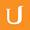## Variation Practice 2 - Visualizing Algebra

• 0:00 - 0:04
And in this problem, a would equal 3. Way to go if you got that one correct.
• 0:04 - 0:09
Since a varies inversely with b, we want to make sure that we put the b quantity
• 0:09 - 0:14
in the denominator. But for c, a varies directly with it. So we want to put that
• 0:14 - 0:17
straight across in the numerator. This is perhaps the trickiest part about
• 0:17 - 0:21
setting up variation problems. And you want to make sure you get it right each
• 0:21 - 0:25
time. Remember, with inverse relationships. They'll appear in opposite
• 0:25 - 0:29
positions, whereas with direct relationships, the variables will appear directly
• 0:29 - 0:34
across from one another. So in my first case, a is 12, b is three, and c is
• 0:34 - 0:39
eight. So I plug in those values. In the second case, we don't know the value of
• 0:39 - 0:44
a, so I leave that written as a in the denominator. But I do know that b2 is
• 0:44 - 0:49
equal to six. And c2 is equal to 4. These are the values of b and c in our
• 0:49 - 0:53
second case. Now that we have this equation, we multiply these two fractions
• 0:53 - 0:59
together to get 48 divided by 12. We cross multiply to get 144 equals 48a, and
• 0:59 - 1:03
finally we divide both sides by 48 to get a is equal to 3.
Cím:
Variation Practice 2 - Visualizing Algebra
Video Language:
English
Team:Udacity
Projekt:
MA006 - Visualizing Algebra
Duration:
01:04Udacity Robot edited Angol subtitles for Variation Practice 2 - Visualizing AlgebraCogi-Admin hozzáadott egy fordítást

# English subtitles

## Felülvizsgálatok Compare revisions

• API
Udacity Robot